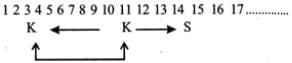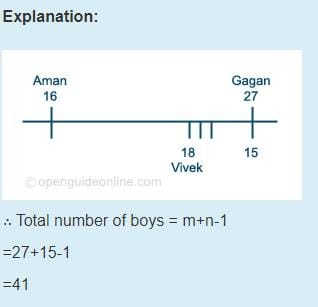# Test: Ranking- 3

## 20 Questions MCQ Test IBPS PO Prelims- Study Material, Online Tests, Previous Year | Test: Ranking- 3

Description
Attempt Test: Ranking- 3 | 20 questions in 15 minutes | Mock test for Banking Exams preparation | Free important questions MCQ to study IBPS PO Prelims- Study Material, Online Tests, Previous Year for Banking Exams Exam | Download free PDF with solutions
QUESTION: 1

Solution:
QUESTION: 2

### Children are in pursuit of a dog whose leash has brokenKrishnarajan is directly behind the dog. Rangarajan is behind Krishnarajan. Natrajan is behind Rangarajan Premrajan is ahead of the dog walking down the street in the opposite direction. As the children and dog pass, Premrajan turns around and joins the pursuit. He runs in behind Rangarajan. Krishnarajan runs faster and is alongside the dog on the left. Rangarajan runs faster and is alongside the dog on the right. Which child is directly behind the dog.

Solution:

1. From the second and the third statements of the passage, the arrangement is:

Dog

Krishnarajan

Rangarajan

Natrajan

2. It is given that Premarajan runs behind Rangarajan.

Dog

Krishnarajan

Rangarajan

Premarajan

Natrajan

Krishnarajan and Rangarajan run faster and reach along side the dog on left and right respectively. The final positions are follows

Krishnarajan Dog
Rangarajan

Premarajan

Natarajan

∴Premarajan is directly behind the dog.

QUESTION: 3

### If in a row, there are 23 persons, then find the position of the person exactly in the middle?

Solution:
QUESTION: 4

30 persons are sitting in a row. Find the number of persons sitting between 9th person from left and 16th person from right.

Solution:

The correct answer is D as we need to count 9th from left and 16th from right too
So
30-9=21

QUESTION: 5

40 persons are sitting in a row. How many persons are sitting between 31st person from left and 25th person from right?

Solution:

position of 25th person from left - 41 - 25 =16.
persons between = 31 - 16 -1 = 14

QUESTION: 6

In a row of 40 girls, when Komal was shifted to her left by 4 places her number from the left end of the row become 10. What was the number of Swati from the right end of the row, if Swati was three places to the right of Komal’s original position?

Solution:

The correct option is B.
On shifting 4 the left Komal is 10th from the left end of the row. Thus, Komal's original position was 14th from the left end.Swati is 3 places to the right of Komal's original positions Clearly, swati is 17 th from the left end. Number of girls to the right of swati =(40−17)=23.
Thus, Swati is 24th from the right end of the row.

QUESTION: 7

Amar is 16th from the left end in a row of boys and Vishal is 18th from the right end. Ganesh is 11th from Amar towards the right and 3rd from Vishal towards the right end. How many boys are there in the row?

Solution:QUESTION: 8

Each of the six poles P, Q, R, S, T and U has a different height. P is taller than only three poles. S is taller than T but shorter than U. U is shorter than P. Q is not the tallest. The second shortest pole is 2 feet tall. The third tallest pole is 5 feet tall.

Q.
Which of the following may be the possible height of pole U?

Solution:

←      Six Poles     →
1   2    3    4   5    6

↓    ↓    ↓     ↓   ↓     ↓

T   S    U    P   Q    R

↓          ↓

2 feet    5 feet

QUESTION: 9

Each of the six poles P, Q, R, S, T and U has a different height. P is taller than only three poles. S is taller than T but shorter than U. U is shorter than P. Q is not the tallest. The second shortest pole is 2 feet tall. The third tallest pole is 5 feet tall.

Q. ​
The tallest pole is 11 feet taller than S. Which of the following may be the possible height of Q?

Solution:
QUESTION: 10

Each of the six poles P, Q, R, S, T and U has a different height. P is taller than only three poles. S is taller than T but shorter than U. U is shorter than P. Q is not the tallest. The second shortest pole is 2 feet tall. The third tallest pole is 5 feet tall.

Q. ​​
Which of the following is true with respect to the given information?

Solution:
QUESTION: 11

In a class of 150, where girls are twice the number of boys,Jatin a boy, ranked thirty fourth from the top in the class. If there are eighteen girls ahead of Jatin, How many boys are after him in rank?

Solution:
QUESTION: 12

In a row of boys, A is fifteenth from left and B is fourth from right end there are three boys between A and B. C is first left of A. What is C’s position from right?

Solution:

The correct option is A.
Total no of boys = position of A from left + Position of B from right + total boys between A and B.
= 15 + 4 + 3 = 22.
Position of A is 15th from left.
Again, left of A is C -
position of C from left = 15-1 = 14
position of c from right = 22+1 - 14 = 9

QUESTION: 13

In a row of 50 students facing north directionRamesh is sixth to the left of Manjit. If Manjit is 28th from left end then how far is Ramesh from left end?

Solution:
QUESTION: 14

Six people A, B, C, D, E and F obtained different marks in an exam. A obtained more marks than only two people. B obtained more marks than D but not the highest. F obtained more marks than only C. The one with second highest marks obtained 480 marks. The one with second lowest marks obtained 350 marks.

Q.
Which ofthe following is true with respect to E as per the given information?

Solution:
QUESTION: 15

Six people A, B, C, D, E and F obtained different marks in an exam. A obtained more marks than only two people. B obtained more marks than D but not the highest. F obtained more marks than only C. The one with second highest marks obtained 480 marks. The one with second lowest marks obtained 350 marks.

Q.
Who amongst the following obtained third highest?

Solution:
QUESTION: 16

Six people A, B, C, D, E and F obtained different marks in an exam. A obtained more marks than only two people. B obtained more marks than D but not the highest. F obtained more marks than only C. The one with second highest marks obtained 480 marks. The one with second lowest marks obtained 350 marks.

Q.
Who amongst the following possibly obtained 400marks.

Solution:
QUESTION: 17

A group of dancers are standing in a line for their performance. Joseph tried to calculate his rank from either side and found that his rank is 19th. Find the total number of dancers standing in that line.

Solution:

Ans: b

Explanation: If we count till Joseph from the left side, then he will be 19 dancers. Similarly, from the right-hand side, the total number of students to Joseph will also be 19. Now adding both and subtracting 1 (dual occurrence of Joseph) we will get = 19 + 19 - 1 = 37.

QUESTION: 18

In a classof 180, where girls are twice the number of boys. Ritesh a boy ranked thirty fourth from the top. If there are eighteen girls ahead of Rupesh how many boys are after him in rank?

Solution:

B is the correct option.No. of boys upto the 34th rank = 34-18 = 16
Total no of boys = 108/2+1 ×=60
∴ number of boys after the rank of Rupesh = 60 - 16 = 44

QUESTION: 19

There are six people A, B, C, D, E and F each having different weight. A is heavier than F but lighter than B. E is heavier than C but lighter than A. B is higher than D. C is not the lighest. The second lighest person weight 52kg while the third lighest is of 64kg.

Q.
Who among the following may be 58 kg in weight?

Solution:

If 2nd lightest person weight is 52kg and the 3rd lightest is of 64kg , then 58 kg person should lie between 52 and 64 but its not possible. So  option c is the correct answer.

QUESTION: 20

There are six people A, B, C, D, E and F each having different weight. A is heavier than F but lighter than B. E is heavier than C but lighter than A. B is higher than D. C is not the lighest. The second lighest person weight 52kg while the third lighest is of 64kg.

Q.
How many persons are heavier than C?

Solution:

The correct option is D.

As per the given information the sequence will be =

B>D>A>E>C>F

So 4 people are heavier than C.Use Code STAYHOME200 and get INR 200 additional OFF Use Coupon Code